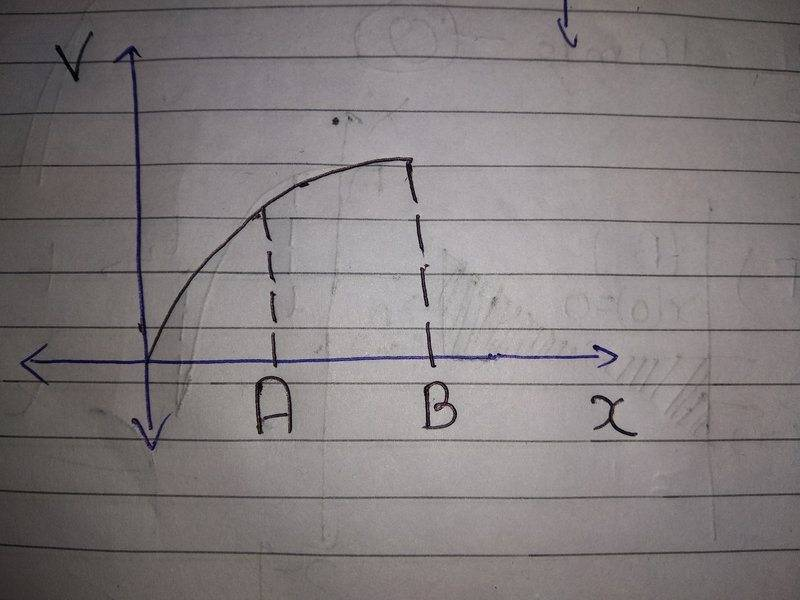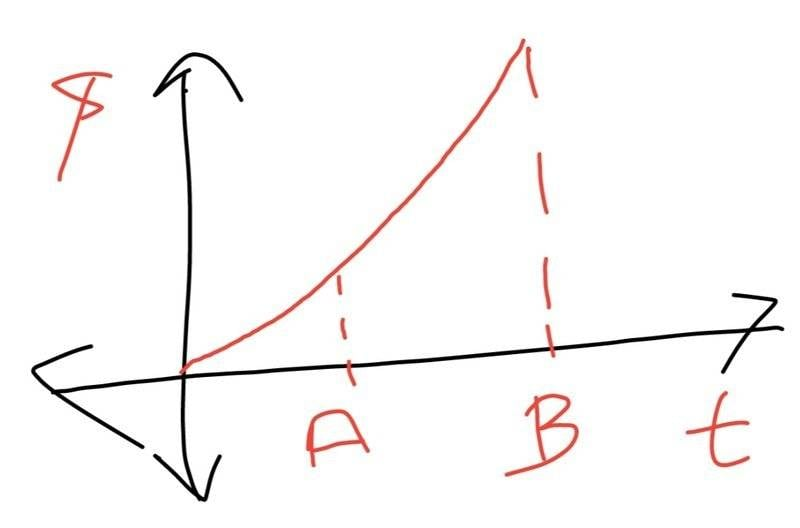# Steps for converting one type of graph to the other?

• navneet9431

Gold Member

## Homework StatementSuppose this the velocity time graph given and we want to plot position time grah from this.
What are the things to be considered while plotting the position time graph from this graph?

2. Homework Equations

The uniform acceleration motion equations.

## The Attempt at a SolutionI think this is what the graph should look like because area under the velocity time cure is continuously increasing.

I will be thankful for any help!

#### Attachments

Last edited:
The position is always increasing = the velocity is always positive, good.
The rate of the increase in position is increasing (the curve turns upwards) = the velocity is increasing, good.
You start with zero velocity, the beginning of the curve could be a bit flatter.
Mapping the rate of this increase accurately is difficult. Towards the end it looks like the velocity approaches a constant, so the position graph should become close to a straight line.

•navneet9431
@navneet9431, the word is "graph" not "grah".

@navneet9431, the word is "graph" not "grah".
I have edited it!

What are the things to be considered while plotting the position time graph from this graph?
Is that the question asked of you, or were you asked to sketch the graph and this is your question to the forum?
If the first, you need to say more about how you arrived at the sketch. Specifically, explain why you have the slope increasing.